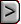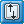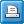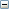﻿ GMG - Geometric Multigrid Solver
Online Guide to MODFLOW

GMG - Geometric Multigrid SolverGMG - Geometric Multigrid SolverGMG - Geometric Multigrid SolverAbbreviation in Name file

GMG

Purpose

The Geometric Multigrid Solver is used to solve the finite difference equations in each step of a MODFLOW stress period.

Documentation

 • Wilson, J.D. and Naff, R.L., 2004, The U.S. Geological Survey modular ground-water model -- GMG linear equation solver package documentation:  U.S. Geological Survey Open-File Report 2004-1261, 47 p.
 • Banta, Edward R., 2006, Modifications to MODFLOW boundary conditions and an adaptive-damping scheme for Picard iterations for a highly nonlinear regional model, in Poeter, Eileen, Hill, Mary, and Zheng, Chunmiao, MODFLOW and More 2006—Managing Ground Water Systems: International Ground Water Modeling Center and Colorado School of Mines, Golden, Colorado, 2006 [Proceedings], p. 596-600.
 • GMG_Damping.pdf

Related Packages

Solvers

Supported in

 • MODFLOW-2000
 • MODFLOW-2005
 • MODFLOW-LGR
 • MF2005-NWT
 • MODFLOW-OWHM

Other Notes

If the Lake package is used, more iterations may be required.

Input Instructions

The GMG solver package has both an inner loop and outer loop. The inner loop is set up for the convergence of the linear problem and is controlled by the parameters RCLOSE and IITER. A nonlinear problem is controlled through the outer loop and uses parameters HCLOSE and MXITER. As a solution of the linear problem is used as an approximate solve for the nonlinear problem, all four parameters are needed when solving a nonlinear problem. The operation of these parameters is further explained in the section titled “Module GMG1AP”.

Input for the GMG package is read from the file that is type ”GMG” in the name file. Free format is used for reading all values on the input list. The GMG data file should contain the following data items:

FOR EACH SIMULATION

Data Set -1

[#Text]

Item -1 is optional—“#” must be in column 1. Item 0 can be repeated multiple times.Text
 An optional [#Text] item can be inserted multiple times before any of the above items; the symbol # must appear in the first column. These optional items are treated as comments. The convergence criteria for the GMG package may look similar to other packages, such as the PCG2 package described in Hill (1990), but their meaning may be quite different. The reader is encouraged to refer back to the “Convergence Criteria” section for more detail.

Data Set 0

RCLOSE IITER HCLOSE MXITERExplanation of variablesRCLOSE
 RCLOSE is the residual convergence criterion for the inner iteration. The PCG algorithm computes the l2norm of the residual and compares it against RCLOSE. Typically, RCLOSE is set to the same value as HCLOSE (see below). If RCLOSE is set too high, then additional outer iterations may be required due to the linear equation not being solved with sufficient accuracy. On the other hand, a too restrictive setting for RCLOSE for nonlinear problems may force an unnecessarily accurate linear solution. This may be alleviated with the IITER parameter or with damping.IITER
 IITER is the maximum number of PCG iterations for each linear solution. A value of 100 is typically sufficient. It is frequently useful to specify a smaller number for nonlinear problems so as to prevent an excessive number of inner iterations.HCLOSE
 HCLOSE is the head change convergence criterion for nonlinear problems. After each linear solve (inner iteration), the maxnorm of the head change is compared against HCLOSE. HCLOSE can be set to a large number for linear problems; HCLOSE is ignored if MXITER=1.MXITER
 MXITER is the maximum number of outer iterations. For linear problems, MXITER can be set to 1. For nonlinear problems, MXITER needs to be larger, but rarely more than 100.

Data Set 1Explanation of variablesDAMP
 DAMP is the value of the damping parameter. For linear problems, a value of 1.0 should be used. For nonlinear problems, a value less than 1.0 but greater than 0.0 may be necessary to achieve convergence. A typical value for nonlinear problems is 0.5. Damping also helps control the convergence criterion of the linear solve to alleviate excessive PCG iterations (see equation (20).IADAMP

IADAMP is a flag that controls adaptive damping. The possible values of IADAMP and their meanings are as follows:

 • If IADAMP = 0, then the value assigned to DAMP is used as a constant damping parameter.
 • If IADAMP = 1, the value of DAMP is used for the first nonlinear iteration. The damping parameter is adaptively varied on the basis of the head change, using Cooley’s method as described in Mehl and Hill (2001), for subsequent iterations.
 • If IADAMP = 2, the relative reduced residual damping method documented in Mehl and Hill (2001) and modified by Banta (2006) is used.

When IADAMP is specified as 2 and the value specified for DAMP is less than 0.5, the closure criterion for the inner iterations (DRCLOSE) is assigned simply as RCLOSE. When DAMP is between 0.5 and 1.0, inclusive, or when IADAMP is specified as 0 or 1, DRCLOSE is calculated according to equation 20 on p. 9 of Wilson and Naff (2004).IOUTGMG

IOUTGMG is a flag that controls the output of the GMG solver. The possible values of IOUTGMG and their meanings are as follows:

 • If IOUTGMG = 0, then only the solver inputs are printed.
 • If IOUTGMG =1, then for each linear solve, the number of PCG iterations, the value of the damping parameter, the l2norm of the residual, and the maxnorm of the head change and its location (column, row, layer) are printed. At the end of a time/stress period, the total number of GMG calls, PCG iterations, and a running total of PCG iterations for all time/stress periods are printed.
 • If IOUTGMG = 2, then the convergence history of the PCG iteration is printed, showing the l2norm of the residual and the convergence factor for each iteration.
 • IOUTGMG =3 is the same as IOUTGMG =1 except output is sent to the terminal instead of the MF2K LIST output file.
 • IOUTGMG =4 is the same as IOUTGMG =2 except output is sent to the terminal instead of the MF2K LIST output file.IUNITMHC

IUNITMHC is a flag and a unit number, which controls output of maximum head change values:

 • If IUNITMHC = 0, maximum head change values are not written to an output file.
 • If IUNITMHC > 0, maximum head change values are written to unit IUNITMHC. Unit IUNITMHC should be listed in the Name file with “DATA” as the file type.
 • If IUNITMHC < 0 or is not present, IUNITMHC defaults to 0.

Data Set 2

ISM ISC [DUP DLOW CHGLIMIT]Explanation of variablesISM

ISM is a flag that controls the type of smoother used in the multigrid preconditioner. The possible values for ISM and their meanings are as follows:

 • If ISM = 0, then ILU(0) smoothing is implemented in the multigrid preconditioner. This smoothing requires an additional vector on each multigrid level to store the pivots in the ILU factorization.
 • If ISM = 1, then Symmetric GaussSeidel (SGS) smoothing is implemented in the multigrid preconditioner. No additional storage is required for this smoother; users may want to use this option if available memory is exceeded or nearly exceeded when using ISM=0. Using SGS smoothing is not as robust as ILU smoothing; additional iterations are likely to be required in reducing the residuals. In extreme cases, the solver may fail to converge as the residuals cannot be reduced sufficiently.ISC

ISC is a flag that controls semicoarsening in the multigrid preconditioner. The possible values of ISC and their meanings are given as follows:

 • If ISC = 0, then the rows, columns and layers are all coarsened.
 • If ISC = 1, then the rows and columns are coarsened, but the layers are not.
 • If ISC = 2, then the columns and layers are coarsened, but the rows are not.
 • If ISC = 3, then the rows and layers are coarsened, but the columns are not.
 • If ISC = 4, then there is no coarsening.

Typically, the value of ISC should be 0 or 1. In the case that there are large vertical variations in the hydraulic conductivities, then a value of 1 should be used (see Remark 9 in “CoarseGrid Correction” section of Wilson and Naff (2004)). If no coarsening is implemented (ISC = 4), then the GMG solver is comparable to the PCG2 ILU(0) solver described in Hill (1990) and uses the least amount of memory.DUP
 DUP is the maximum damping value that should be applied at any iteration when the solver is not oscillating; it is dimensionless. An appropriate value for DUP will be problem-dependent. For moderately nonlinear problems, reasonable values for DUP would be in the range 0.5 to 1.0. For a highly nonlinear problem, a reasonable value for DUP could be as small as 0.1. When the solver is oscillating, a damping value as large as 2.0*DUP may be applied.DLOW
 DLOW is the minimum damping value to be generated by the adaptive-damping procedure; it is dimensionless. An appropriate value for DLOW will be problem-dependent and will be smaller than the value specified for DUP. For a highly nonlinear problem, an appropriate value for DLOW might be as small as 0.001. Note that the value specified for the next variable, CHGLIMIT, could result in application of a damping value smaller than DLOW.CHGLIMIT
 CHGLIMIT is the maximum allowed head change at any cell between outer iterations; it has units of length. The effect of CHGLIMIT is to determine a damping value that, when applied to all elements of the head-change vector, will produce an absolute maximum head change equal to CHGLIMIT.

Data Set 3

RELAX

This item is read only if ISC = 4RELAX
 RELAX is a relaxation parameter for the ILU preconditioned conjugate gradient method. The RELAX parameter can be used to improve the spectral condition number of the ILU preconditioned system. The value of RELAX should be approximately one. However, the relaxation parameter can cause the factorization to break down. If this happens, then the GMG solver will report an assembly error and a value smaller than one for RELAX should be tried. This item is read only if ISC = 4.